# What is confidence interval statistics

## Confidence interval for expected value, variance and median

If you have collected a suitable sample for your estimate of the population, you can use it to make estimates for theparameter of the population. Such parameters are e.g. B. the expected value, the Variance or the Median.

These values ​​are point estimates. There is very little probability that they exactly match the parameters of the population. This is true even if they all have statistically desirable properties. It is therefore interesting to determine in which number range the true parameter is located.

### What is a confidence interval?

A confidence interval or confidence interval is an interval range determined from a sample with the help of estimation theory. The parameters of the population then lie in this range with a certain probability. The limits of the interval are random variables.
In the course of an investigation of the spending behavior of students in Germany, for example, you drew a suitable sample of n = 200. You asked about food expenditure as follows: "How many euros do you spend on food per month?"
The evaluation of the answers then resulted in the following estimated values ​​for the true parameters:

You are now looking for symmetrical intervals around these estimated values, in which the true parameter values ​​lie with a probability of 95%.

### Confidence interval for the expected value E (X)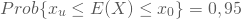With large samples, for example from n = 100, you can regard E (X) as normally distributed according to the Central Limit Theorem. You then transform everything into standard normally distributed values.
The standardized limits of the 95% confidence interval then result as z-values ​​of the standard normal distribution at the points 2.5% and 97.5%: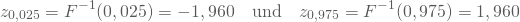From these standardized values ​​you can then calculate the interval limits by reversing the standardization: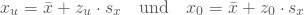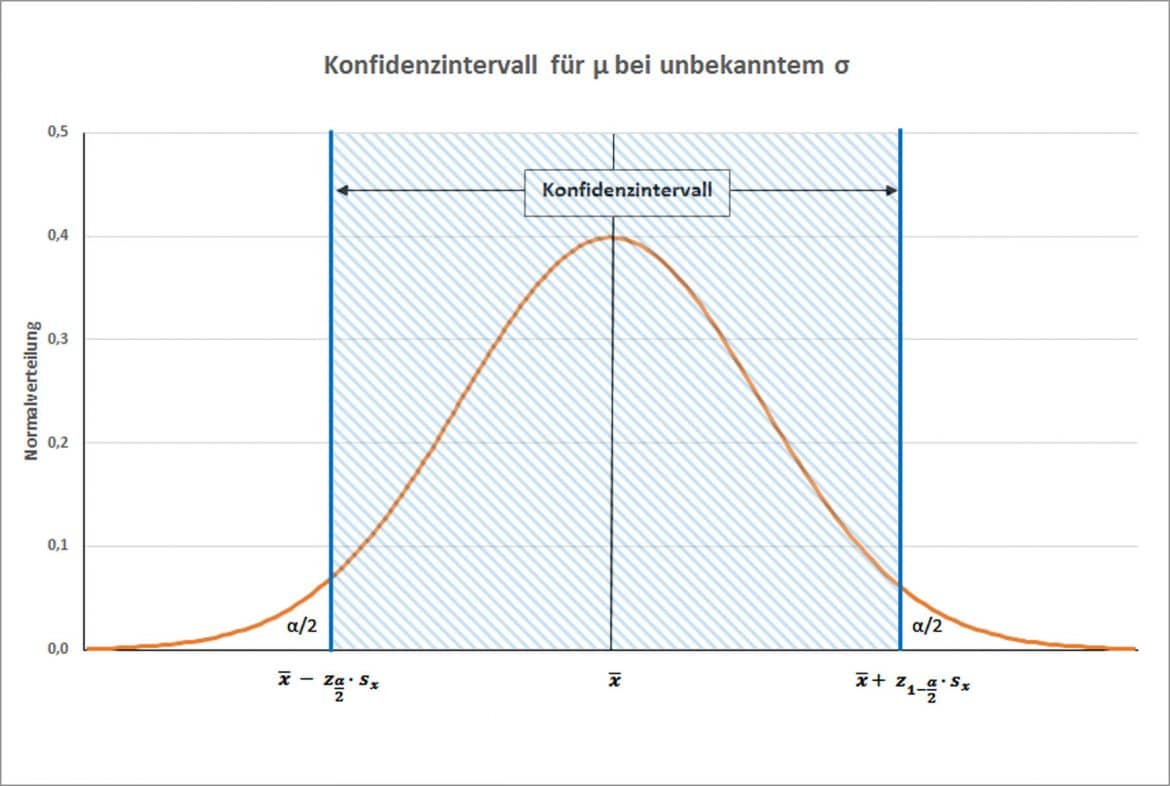Inserting the numbers then results in the lower and upper limits of your confidence interval.There is a 95% probability that the data provided a confidence interval that was true Average contains. The expected value of the monthly expenses of students for food is therefore between 147.80 € and 226.20 €.
For small samples with n <100, on the other hand, you cannot assume a normal distribution. Then the standardized random variable follows a t-distribution with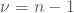Degrees of freedom. To calculate the limits of the confidence interval, the z-values ​​are then replaced by the corresponding values ​​of the t-distribution.

### Confidence interval for the variance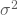Here, instead of the normal distribution, you use the quotient Sample variance and population variance one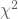Distribution withDegrees of freedom follows.

So it results: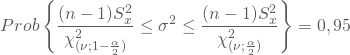You put your sample estimates and the values ​​of the distribution into this general formula. This gives you the lower and upper limits of the confidence interval for the variance: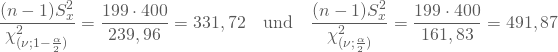The confidence interval with the limits 331.72 and 491.87 that the sample delivered contains your true variance with a probability of 95%.

### Confidence interval for the median

In the case of one large sample With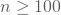the distribution of X and E (X) can be viewed as asymptotically normally distributed. The normal distribution is symmetrical. This means that the expected value and median coincide. The confidence interval for the median is then identical to that for the expected value.

In the case of one small sample and unknown distribution of the population, statistical programs still offer options. In this way, you can usually determine asymmetrical confidence intervals for the median using the bootstrapping method.